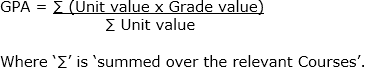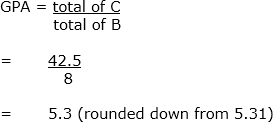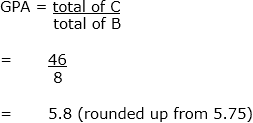Grade Point Average (GPA) Calculation Schedule

1 Purpose

To establish the method of calculation of a Student's Grade Point Average (GPA).

2 Scope

This schedule must be read in conjunction with the Assessment Procedure and is subordinate to it. This schedule applies to all Students and is also used for consideration of Medals and Awards With Distinction and should be read in conjunction with the Award Eligibility and Graduation Policy.

3 Schedule

Table 1 below shows the mapping of final mark to equivalent Final Grade and to Grade Point calculation.

Table 1: Determination of Final Grades

 Final mark out of 100 Equivalent Final Grade Grade Point At least 85 High Distinction (HD) 7 At least 75 but less than 85 Distinction (A) 6 At least 65 but less than 75 Credit (B) 5 At least 50 but less than 65 Pass (C) 4 At least 50 Ungraded Pass (UP)* Not Applicable* At least 50 Pass - Not Contributing to GPA (PC) Not applicable Less than 50 Fail (F, FNP, FNS, FNC, FLW) 1.5 Less than 50 Ungraded Fail (UF)* Not applicable* Less than 50 Fail - Not Contributing to GPA (FC) Not applicable

*Only available within the non-stratified system in which the Grades of UP and UF do not contribute towards a Student's GPA.

The current and historic codes and numerical values of Grades are provided in Table 2 below.

Table 2: Numerical Values of Grades

 GPA on 7 point scale as used by USQ 7 6 5 4 3 1.5 Equivalent GPA on the 4 scale 4 3.5 2.5 1.5 1 .5 2010 onwards HD A B C D F Semester 2, 2007 to 2009 HD A B C LP F 1994 to Semester 1, 2007 HD A B C D F

3.3 Calculation of Grade Point Averages

1. The GPA may be:
1. cumulative GPA, calculated over all Courses undertaken within the Award Program;
2. calculated over a specified number of Courses or all Courses at or above a specified level; or
3. calculated over the Courses in an approved Major/Academic Plan.
4. for Medals and for With Distinction as per item 3.4 in this schedule.
2. The GPA can be expressed mathematically as:3. The above calculation results in a number (GPA) between 1.5 and 7.

Example 1:

 A X B = C Course Grade Numerical value of Grade x Number of Units = Total ABC1000 A 6 X 1 = 6 ABC1001 C 4 X 1 = 4 ABC1002 B 5 X 1 = 5 ABC1003 F 1.5 X 1 = 1.5 ABC1004 HD 7 X 2 = 14 ABC1005 A 6 X 2 = 12 TOTALS 8 42.5Example 2:

 A X B = C Course Grade Numerical value of Grade X Number of Units = Total XYZ1000 A 6 X 1 = 6 XYZ1001 C 4 X 1 = 4 XYZ1002 B 5 X 1 = 5 XYZ1003 B 5 X 1 = 5 XYZ1004 HD 7 X 2 = 14 XYZ1005 A 6 X 2 = 12 TOTALS 8 463.4 Calculation of GPA for Medals and for With Distinction

The determination of eligible Courses to be included in the calculation of the GPA for Medals and for With Distinction is as follows:

1. A Course undertaken at the University and within the relevant Award Program.
2. A Course for which there has been a Transfer of Grade from an incomplete Award Program.
3. A Course from an Award Program that has been accredited to allow a Transfer of Grade from a completed Award Program to the Award Program in which the Student is enrolled.
4. The criteria for the relevant Courses will be determined via the applicable specification for the rationale for calculation, e.g. if the calculation is for a University Medal the criteria for the relevant Courses is specified in the Medals and Awards With Distinction Schedule.
5. Where a Student has received Credit from an external institution (including Cross-institutional Study), only those Units completed at the University will be included.
6. Where a Student has completed more than the maximum Units required for the Award Program, only the approved Units will be counted.
7. Where, through an administrative error, a Student has passed the same Course twice, the Grade of the first completion, not the highest Grade, will be utilised.
8. Ungraded passes are not included in the calculation of a GPA.
9. The GPA for Medals and for With Distinction will be calculated to one decimal place, that is, a second decimal place of X1 - X4 will be rounded down, a second decimal place of X5 - X9 will be rounded up. For example, 6.44 would round to 6.4, and 6.48 would round to 6.5.

Nil.